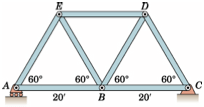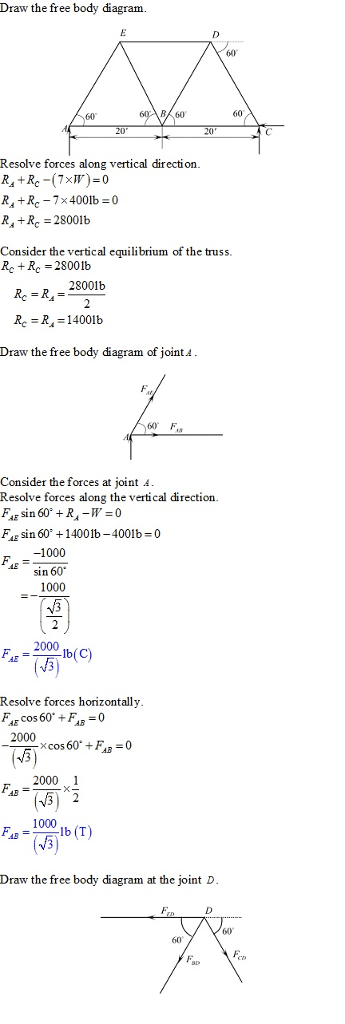# Each member of the truss is a uniform 20-ft bar weighing 400 lb. Calculate the average tension or compression in each member due to the weights of the members

Question-AnswerCategory: Engineering MechanicsEach member of the truss is a uniform 20-ft bar weighing 400 lb. Calculate the average tension or compression in each member due to the weights of the members

Each member of the truss is a uniform 20-ft bar weighing 400 lb. Calculate the average tension or compression in each member due to the weights of the members.